# Off-Axis Conic Surfaces for Non-Axisymmetric Surface Generation

A traditional single point diamond turning (SPDT) lathe describes a curve in space using two axes of motion. The formation of the curve is between the centerline of the work piece to be machined and the cutting edge of a diamond tool. A 3D surface of revolution is generated by the rotation of the work piece through the cutting action. The simplicity of these motions has generally limited the SPDT process to generate axisymmetric surfaces.

## Four Axis SPDT

The advent of four axis SPDT enables the generation of non-axisymmetric surfaces. This tool has two extra motions in addition to the orthogonal two axes of motion (x and z axes) featured in a SPDT lathe. The work holding spindle (f axis) is encoded to create the first additional motion, allowing the machine tool controller to access the work piece’s angular position during machining.

As an additional linear axis, the fourth axis of motion (z' axis) holds the SPDT tool and allows extremely rapid positioning even with a limited range of motion. It is possible to generate a non-axisymmetric surface through coordination of the motion of the tool as a function of the angular position of the work piece.

Off-axis segments can be machined for large mosaic mirrors using four axis SPDT. Traditionally, in SPDT of these surfaces, the segment had to be positioned at the appropriate distance from the work holding spindle axis, and the surface was machined as a portion of an entire parent surface of revolution.

This method limits how far the segment is off-axis from the parent axis to the maximum machine tool swing geometry. This limitation is not based on the segment’s physical size but mainly on the distance that the segment is off-axis. For instance, large machine tools are required even for small segments in case they are very far off-axis. The use of a four axis approach for machining these surfaces allows positioning of the segments with the appropriate off-axis surfaces formed by four axis non-axisymmetric machining.

The surface geometry description of these segments is an aspect of this four axis approach to generate non-axisymmetric surfaces. This article discusses the geometry of off-axis conic surfaces in a geometrically precise manner and can provide the rapid "real-time" solution demanded by four axis positioning at high rotational speeds. This article also reviews considerations minimizing the range of motion of the fourth axis by illustrating the geometries of the off-axis segments of the primary mirror of the Keck Telescope.

## Off-Axis Surface Geometry

A sagitta equation describes most aspheric off-axis surfaces in a coordinate system referenced to the parent surface: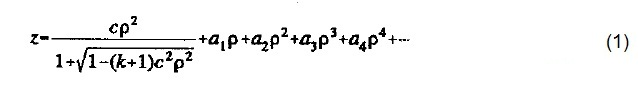Equation 1 defines a conic surface of revolution changed by simple polynomial terms in a cylindrical coordinate system, where the origin of the coordinate system is in coincidence with the vertex of the aspheric surface. The sagitta axis is the z axis and is collinear with the axis of rotation of the asphere. It is necessary to describe the section geometry in a polar coordinate system that is centered on the off-axis surface for the non-axisym metric generation of an off-axis section.

The solution of transcendental equations is required for the modified general aspheric surface. It is possible to solve the case of the general conic of revolution in closed form, which will be taken into account here. The possibility of coordinating the required motions in a "real time" environment in a four-axis control system is one advantage of a closed form description.

Literature for surfaces created by deformation techniques describes approximate representation of off-axis conic surfaces of revolution. Descriptions are also available of approximations for general non-axisymmetric surface fabrication on the basis of Zemike polynomials. The off-axis paraboloid with limitations on orientation has also been described by Thompson. The derivation shown in this article for the general off axis conic surface that is tilted in the meridional plane at an arbitrary angle is based on Thompson’s method.

Thompson's method is divided into four sections. First is the off axis geometry described in the parent conic surface’s coordinate system, with the off-axis segment’s center along the x-axis. The description is then converted to a second coordinate system with its origin is in coincidence with the center of the off-axis segment. This is followed by rotation of the description in the meridional plane to a third coordinate system. The last section is the conversion of this description from a Cartesian form to a cylindrical representation. The coordinate systems used are shown in Figure 1.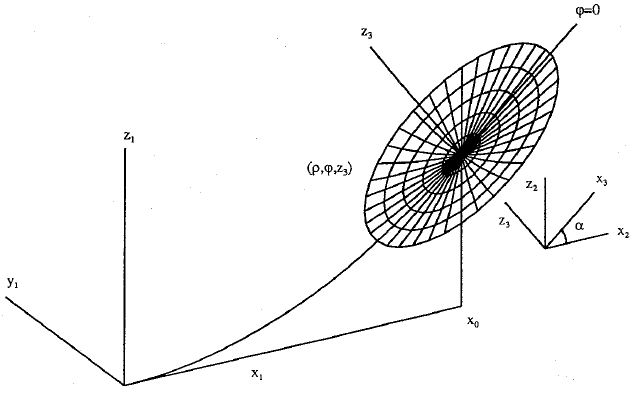Figure 1. Off-axis geometry

The derivation starts with the description of the off-axis conic surface in the parent surface of revolution’s coordinate system. This initial description is expressed in Equation 2 as follows: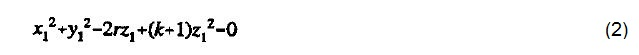where r = Paraxial radius of curvature of the parent conicoid and k = Conic constant.

Equation set 3 can be used to create a second coordinate system by selecting a point (x0, 0, z0) on the off-axis surface a second coordinate system. This is used to translate the origin from the parent vertex to this new point. Direct substitution allows a new description of the centered off-axis surface to be written. When simplified, this description then provides Equation 4: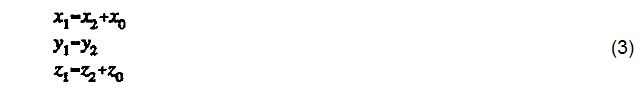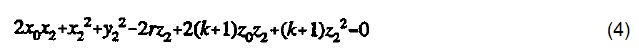The off-axis centered system is rotated through a meridional angle (α) using Equation set 5 to create a third coordinate system.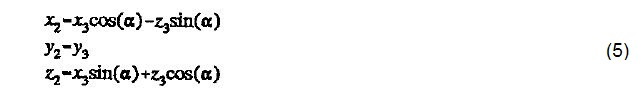Following grouping of like terms and simplification, the description of the off- axis surface takes the form of a quadratic in the new sagitta direction z3 (Equation 6):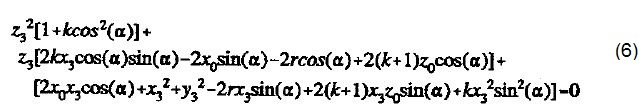Equation set 7 is used for the final transformation of coordinate systems from Cartesian to cylindrical, yielding the quadratic sagitta description in the required final orientation (Equation 8).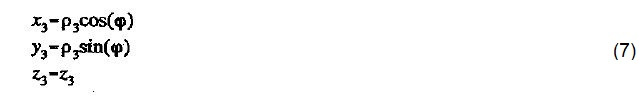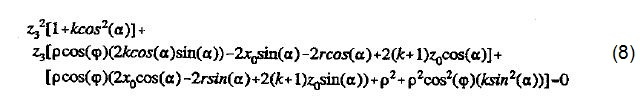The solution takes the form of Equation 9 following considerable simplification: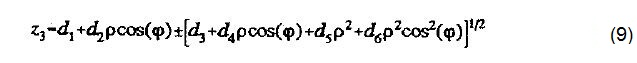The choice of this form allows the dependence of z3 on ρ and φ to be assessed. The new constants generated are expressed in Equations 10 through 15.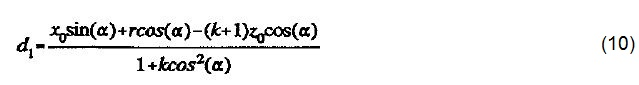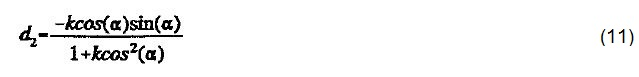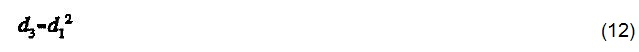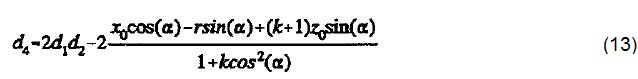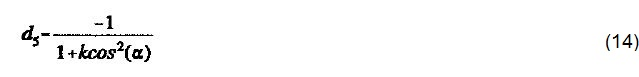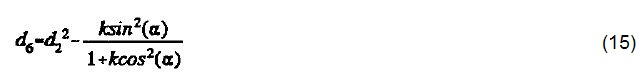There are very few restrictions on the application of this form. The denominator term (1+kcos2(α)) is common in each constant and therefore the form fails when the value of the denominator term is zero. This can occur for hyperboloid segments that are tilted at specific angles, and for the exclusive case of a tilt-free paraboloid. The sign of this denominator term determines the choice of sign for the radical in Equation 9. When the amount below the radical is negative, the form fails again. This occurs when the ρ value goes beyond the range of the conicoid.

## Factors Minimizing Fourth Axis Motion

This description of the off-axis conic surface is suitable for manufacturing schemes using only three axes of motion (x,z,φ). However, the required separation of sagitta motions (z from z') for a four axis machining scheme is not provided by this description. Due to the limited motion range of the fourth axis, the total sagitta motion (z3) has to be divided in a fashion that reduces the fourth axis movement.

This can be achieved by choosing an optimum baseline of motion for the slow z axis and applying the motion of the fast z' axis to generate the required non-axisymmetric motion. Besides selecting an optimum baseline, an optimum meridional tilt angle (a) needs to be selected to reduce the fourth axis motion.

## Illustrative Example

Tessellation of the Keck Telescope primary mirror is illustrated in Figure 2 in order to explain the significance of baseline subtraction effect, and the selection of an optimum tilt angle in reducing fourth axis motion. It is possible to group the 36 off-axis segments of the mirror into six distinct surface shapes. Mirror segments of type 4 and 5 are the right and left hand versions of the same geometry, respectively.

The outline of each projected section is hexagonal when viewed along the rotational axis of the hyperboloid primary. The length of the edges of each hexagon is 0.90 m. The Ritchey-Chretien telescope’s primary mirror has a paraxial radius of curvature of 34.974 meters and a conic constant of -1.003683. Here, the geometry of the sixth segment group is presented as it is the most non- axisymmetric.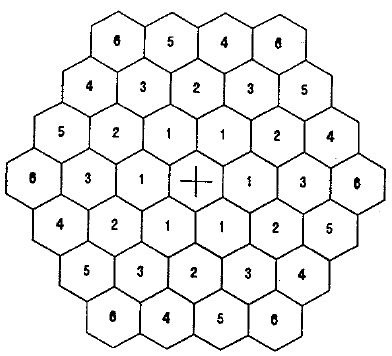Figure 2. Keck Primary Mirror Tessellation

The slope of the surface at the off-axis center point is the first estimate for the optimized meridional tilt angle as observed in the coordinate system of the original parent surface. This estimate is likely to reduce the non- axisymmetric tool motion near the center point but cannot optimize the non- axisymmetric motion at any radial distance away from that point.

The overall non-axisymmetric geometry is reduced by selecting a tilt angle balancing the figure in the meridional plane at the extreme radial positions. The variation between the optimum slope and the original parent slope for the Keck segments are within a few seconds of the arc. The reduction for the optimized tilt over the original slope is typically 20% due to the effect on fourth axis motion.

An anamorphically distorted view of the fourth axis motions for the outside segment of the Keck telescope segment with an optimized tilt angle, and with the slope of the parent conic used, is presented in Figure 3. The wire diagrams are for the minimum inscribed rotational swing needed to create the surfaces. The baseline applied to produce these figures has the z-axis set to the value of z3 at φ=0 for each value of ρ.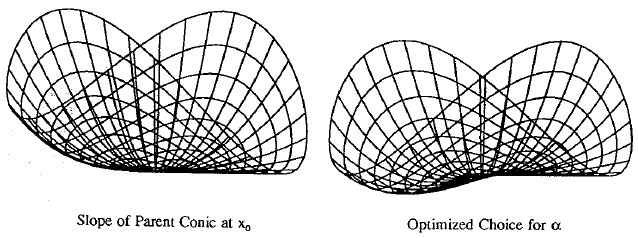Figure 3. Z' fourth axis motion - outside Keck segment (φ=0° baseline subtracted)

Table 1 summarizes the tilt correction from the parent slope applied to optimize the six geometries of the Keck primary mirror.

Table 1. Four Axis Parameters for the keck telescope primary Mirror

row center position x0 tilt correction δα. fourth axis motion z'
1 1558.8 mm -3.10 arc sec 0.0235 mm
2 2700.0 mm -5.31 arc sec 0.0701 rnm
3 3117.7 mm -6.10 arc sec 0.0932 mm
4 4124.3 mm -7.93 arc sec 0.1617 mm
5 4124.3 mm -7.93 arc sec 0.1617 mm
6 4676.5 mm -8.88 arc sec 0.2067 mm

The selection of an optimum baseline is simple. The sagitta motion (z3) has a minimum and maximum value for each radial position. The range of the fourth axis motion is kept centered about a single position by choosing the baseline to be the mean of the minimum and maximum values. Two views of the outside keck segment with both the optimum tilt angle and optimum baseline subtracted are depicted in Figure 4.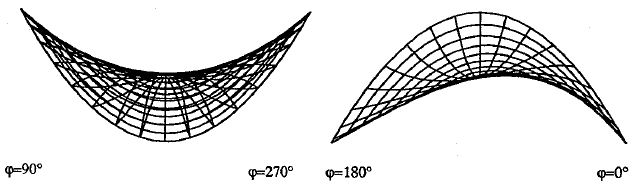Figure 4. Z' fourth axis motion - outside Keck segment (optimized baseline subtracted)

The left image shows the wire frame model, which is the surface as observed from the parent axis and on the right from the orthogonal perspective. Table 1 lists values for the optimized fourth axis motions for all six Keck geometries. The range of fourth axis motion required is very less when compared to the dimensions of all the segments themselves.

## Conclusion

This article presented the derivation of the equations describing the general off-axis conic surface. The derived equations can be applied for the four axis generation of these surfaces in a non-axisymmetric manner. This article also reviewed the factors minimizing the range of motion of the fourth axis.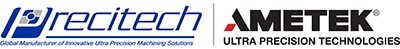This information has been sourced, reviewed and adapted from materials provided by Precitech.

## Citations

• APA

AMETEK Precitech. (2019, May 03). Off-Axis Conic Surfaces for Non-Axisymmetric Surface Generation. AZoM. Retrieved on October 23, 2020 from https://www.azom.com/article.aspx?ArticleID=11917.

• MLA

AMETEK Precitech. "Off-Axis Conic Surfaces for Non-Axisymmetric Surface Generation". AZoM. 23 October 2020. <https://www.azom.com/article.aspx?ArticleID=11917>.

• Chicago

AMETEK Precitech. "Off-Axis Conic Surfaces for Non-Axisymmetric Surface Generation". AZoM. https://www.azom.com/article.aspx?ArticleID=11917. (accessed October 23, 2020).

• Harvard

AMETEK Precitech. 2019. Off-Axis Conic Surfaces for Non-Axisymmetric Surface Generation. AZoM, viewed 23 October 2020, https://www.azom.com/article.aspx?ArticleID=11917.